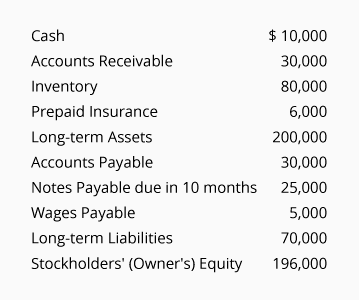# Financial Ratios (Practice Quiz)

For multiple-choice and true/false questions, simply press or click on what you think is the correct answer. For fill-in-the-blank questions, press or click on the blank space provided.

1. 1.

Which of the following is not a current asset account?

Inventory
Prepaid Insurance
Fixtures
2. 2.

Current asset MINUS current liabilities is the

Current Ratio
Net Worth
Working Capital
3. 3.

Current assets DIVIDED BY current liabilities is the

Current Ratio
Net Worth Ratio
Working Capital
4. 4.

The quick ratio EXCLUDES which of the following accounts?

Accounts Receivable
Inventory
Cash
5. Use the following information to answer items 5 - 7:
At December 31 a company's records show the following information:6. 5.

The company's working capital is

\$60,000
\$66,000
\$196,000
7. 6.

The company's current ratio is

1.0 : 1
2.0 : 1
2.1 : 1
8. 7.

The company's quick ratio is

0.7 : 1
1.0 : 1
2.0 : 1
9. Use the following information to answer items 8 - 11:
For its most recent year a company had Sales (all on credit) of \$830,000 and Cost of Goods Sold of \$525,000. At the beginning of the year, its Accounts Receivable were \$80,000 and its Inventory was \$100,000. At the end of the year, its Accounts Receivable were \$86,000 and its Inventory was \$110,000.

10. 8.

The inventory turnover ratio for the year was

4.8
5.0
7.9
11. 9.

The accounts receivable turnover ratio for the year was

6.3
7.5
10.0
12. 10.

On average how many days of sales were in Accounts Receivable during the year?

27
37
49
13. 11.

On average how many days of sales were in Inventory during the year?

14
46
73
14. Use the following information for items 12 and 13:
A company's net income after tax was \$400,000 for its most recent year. The company's income statement included Income Tax Expense of \$140,000 and Interest Expense of \$60,000. At the beginning of the year the company's stockholders' equity was \$1,900,000 and at the end of the year it was \$2,100,000.

15. 12.

What is the times interest earned for the company?

6.7
9.0
10.0
16. 13.

What is the after-tax return on stockholder's equity for the year?

20%
25%
30%
17. 14. The debt to equity ratio is computed as:  (Total Liabilities ÷ Total
__________
) : 1
18. 15.

Which of the following will likely have the reported amounts on the balance sheet closest to their current value?

Current Assets
Long-term Assets
Stockholders' Equity
19. 16.

A corporation's excellent reputation will be listed among the corporation's assets on its balance sheet.

True
False
20. 17.

The current market value of a corporation is approximately the amount reported on the balance sheet as stockholders' equity.

True
False
21. 18.

Free cash flow is the cash provided by operating activities minus the cash used by financing activities.

True
False
22. 19.

The quality of a manufacturer's earnings are suspect when its net income is more than the cash flow from which activities?

Operating
Investing
Financing
23. 20. A balance sheet which reports percentages of total assets instead of dollar amounts is referred to as a
__________
-
__________
balance sheet

Want more practice questions?